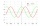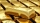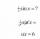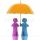# Square (second power, quadratic) + power - math problems

1. The ticketsThe tickets to the show cost some integer number greater than 1. Also, the sum of the price of the children's and adult tickets, as well as their product, was the power of the prime number. Find all possible ticket prices.
2. Substitution methodSolve goniometric equation: sin4 θ - 1/cos2 θ=cos2 θ - 2
3. Precious metalsIn 2006-2009, the value of precious metals changed rapidly. The data in the following table represent the total rate of return (in percentage) for platinum, gold, an silver from 2006 through 2009: Year Platinum Gold Silver 2009 62.7 25.0 56.8 2008 -41.3
4. Cube surface and volumeFind the surface of the cube with a volume of 27 dm3.
5. Find the 2Find the term independent of x in the expansion of (4x3+1/2x)8
6. CubeOne cube has edge increased 5 times. How many times will larger its surface area and volume?
7. Cube 6Volume of the cube is 216 cm3, calculate its surface area.
8. Chord ABWhat is the length of the chord AB if its distance from the center S of the circle k(S, 92 cm) is 10 cm?
9. SaleIf the product twice price cut by 25%, what percentage was price cut in total?
10. Rectangular cuboidThe rectangular cuboid has a surface area 5334 cm2, its dimensions are in the ratio 2:4:5. Find the volume of this rectangular cuboid.
11. PoolIf water flows into the pool by two inlets, fill the whole for 8 hours. The first inlet filled pool 6 hour longer than second. How long pool take to fill with two inlets separately?
12. PowersIs true for any number a,b,c equality:? ?
13. BinomialsTo binomial ? add a number to the resulting trinomial be square of binomial.
14. Widescreen monitorComputer business hit by a wave of widescreen monitors and televisions. Calculate the area of ​​the LCD monitor with a diagonal size 20 inches at ratio 4:3 and then 16:9 aspect ratio. Is buying widescreen monitors with same diagonal more advantageous th
15. Circle chordWhat is the length d of the chord circle of diameter 51 mm, if the distance from the center circle is 19 mm?
16. Triangle SASCalculate the area and perimeter of the triangle, if the two sides are 51 cm and 110 cm long and angle them clamped is 130 °.

Do you have an interesting mathematical word problem that you can't solve it? Enter it, and we can try to solve it.

We will send a solution to your e-mail address. Solved examples are also published here. Please enter the e-mail correctly and check whether you don't have a full mailbox.

Please do not submit problems from current active competitions such as Mathematical Olympiad, correspondence seminars etc...